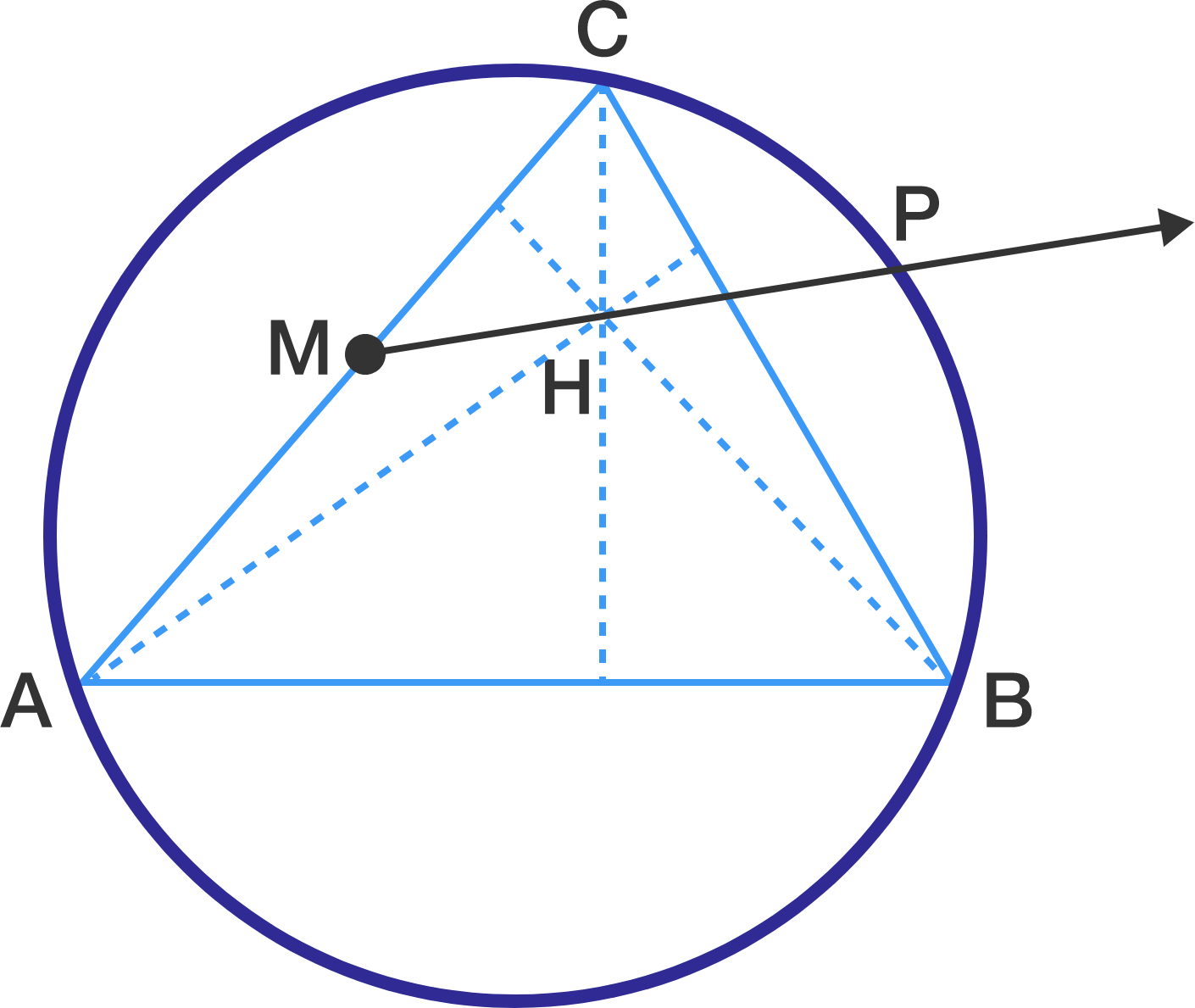# Relating The Orthocenter To The Circumcircle

Geometry Level 3Let $ABC$ be an acute triangle with $AB > BC$. Let $H$ be the orthocenter (intersection of altitudes) of this triangle and $M$ be the midpoint of $AC$. The ray $\overrightarrow{M H}$ intersects the circumcircle of triangle $ABC$ at point $P$, where $P$ belongs to the minor arc $BC$. It is known that $\angle ABP=90^{\circ}$, $MH=5$ and $HP=16$. Find the length of $BC$.

×

Problem Loading...

Note Loading...

Set Loading...